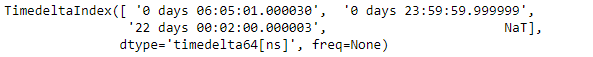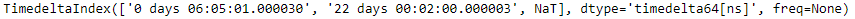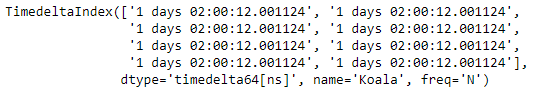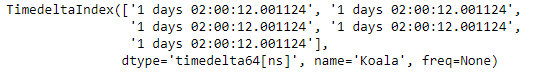Related Articles
Python | Pandas TimedeltaIndex.delete
• Last Updated : 28 Dec, 2018

Python is a great language for doing data analysis, primarily because of the fantastic ecosystem of data-centric python packages. Pandas is one of those packages and makes importing and analyzing data much easier.

Pandas` TimedeltaIndex.delete()` function make a new DatetimeIndex with passed location deleted. The function can delete more than one locations at a time. It takes the index of the label to be deleted as the parameter.

Syntax : TimedeltaIndex.delete(loc)

Parameters :
loc : Indicate which sub-arrays to remove.

Return : new_index : TimedeltaIndex

Example #1: Use `TimedeltaIndex.delete()` function to delete the passed index from the TimedeltaIndex object.

 `# importing pandas as pd``import` `pandas as pd`` ` `# Create the TimedeltaIndex object``tidx ``=` `pd.TimedeltaIndex(data ``=``[``'06:05:01.000030'``, ``'+23:59:59.999999'``,``                                       ``'22 day 2 min 3us 10ns'``, ``None``])`` ` `# Print the TimedeltaIndex object``print``(tidx)`

Output :Now we will use the `TimedeltaIndex.delete()` function to delete the value at 1st index.

Note : The index value begins from 0.

 `# delete the passed index``tidx.delete(``1``)`

Output :As we can see in the output, the `TimedeltaIndex.delete()` function has deleted the passed index and has returned a new object.

Example #2: Use `TimedeltaIndex.delete()` function to delete the passed index from the TimedeltaIndex object.

 `# importing pandas as pd``import` `pandas as pd`` ` `# Create the first TimedeltaIndex object``tidx ``=` `pd.TimedeltaIndex(start ``=``'1 days 02:00:12.001124'``, periods ``=` `8``,``                                             ``freq ``=``'N'``, name ``=``'Koala'``)`` ` `# Print the TimedeltaIndex object``print``(tidx)`

Output :Now we will use the `TimedeltaIndex.delete()` function to delete the value at the passed index.

Note : The index value begins from 0.

 `# delete the passed indexes``tidx.delete([``2``, ``3``, ``4``])`

Output :As we can see in the output, the `TimedeltaIndex.delete()` function has deleted the passed indexes and has returned a new object.

Attention geek! Strengthen your foundations with the Python Programming Foundation Course and learn the basics.

To begin with, your interview preparations Enhance your Data Structures concepts with the Python DS Course.

My Personal Notes arrow_drop_up